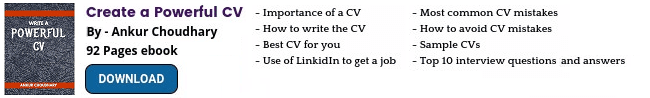Preparation of Molar and Normal Solutions : Pharmaceutical Guidelines -->
 Editable Pharmaceutical Documents in MS-Word Format

# Preparation of Molar and Normal Solutions

Learn how to prepare normal and molar solutions for analysis and formula to calculate the weight to be used for solution preparation.
Molar and normal solutions are used in the chemical analysis of raw materials. A specific amount of chemical (solute) is dissolved to obtain the solution of required concentration (molar or normal solutions).

## A. Molar Solutions

Molar solutions are prepared by dissolving the gram molecular weight of the solute making 1 liter of solution. It means, to prepare 1 liter solution, we have to dissolve the solute equal to the molecular weight of the solute in grams.

Example 1
Preparation of 1M solution of H2SO4
Molecular weight of H2SO4 = 2+32+64 = 98
We require 98 grams of H2SO4 (100%) to prepare 1 liter of 1M H2SO4 solution.

Example 2
Preparation of 1M solution of NaOH
Molecular weight of NaOH =23+16+1 = 40
We require 40 gm of NaOH (100%) to prepare 1 liter of 1M NaOH solution.

## B. Normal Solutions

Normal solutions are prepared by dissolving gram equivalent weight of solute making 1 litre of solution. It means, to prepare 1 liter solution, we have to dissolve the solute equal to the equivalent weight of the solute in grams.
Equivalent weight of any chemical is calculated by dividing the molecular weight with its valence. Valency is the number of ions that can be displaced.
Equivalent weight = Molecular weight / Valency

Example
Preparation of 1N solution of H2SO4
Molecular weight of H2SO4 = 2+32+64 = 98
Valency of H2SO4 = 2 (2 hydrogen ions)
Equivalent weight = 98/2 = 49
We require 49 gm of H2SO4 (100%) to prepare 1 litre of 1N H2SO4 solution.

Note: If the solute is not 100% pure then calculate the required quantity using the following formula.
Actual quantity (gm) = Theoretical quantity (gm) x 100
Purity (%)
For example you require 98 gm of H2SO4 to prepare 1 liter of 1M H2SO4 but the actual purity of H2SO4 is 98% therefore calculate the actual quantity as below.
Actual quantity (gm) = (98 x 100)/98  = 100 gm## Popular Categories

QA SOPs QC SOPs Micro SOPs HVAC Production SOPs Stores SOPs Checklists Maintenance SOPs HPLC Sterile GLP Validation Protocols Water System GDP Regulatory Maintenance Calibration Warning Letters

## DOCUMENTS

PHARMACEUTICAL DOCUMENTS

Editable Pharmaceutical Documents in MS-Word Format. Ready to use SOPs, Protocols, Master Plans, Manuals and more...

View

## Discussion - Latest Topics

Show All ❭❭Pharmaguideline Forum